# CSI Feedback with Autoencoders

This example shows how to use an autoencoder neural network to compress downlink channel state information (CSI) over a clustered delay line (CDL) channel. CSI feedback is in the form of a raw channel estimate array.

### Introduction

In conventional 5G radio networks, CSI parameters are quantities related to the state of a channel that are extracted from the channel estimate array. The CSI feedback includes several parameters, such as the Channel Quality Indication (CQI), the precoding matrix indices (PMI) with different codebook sets, and the rank indicator (RI). The UE uses the CSI reference signal (CSI-RS) to measure and compute the CSI parameters. The user equipment (UE) reports CSI parameters to the access network node (gNB) as feedback. Upon receiving the CSI parameters, the gNB schedules downlink data transmissions with attributes such as modulation scheme, code rate, number of transmission layers, and MIMO precoding. This figure shows an overview of a CSI-RS transmission, CSI feedback, and the transmission of downlink data that is scheduled based on the CSI parameters.The UE processes the channel estimate to reduce the amount of CSI feedback data. As an alternative approach, the UE compresses and feeds back the channel estimate array. After receipt, the gNB decompresses and processes the channel estimate to determine downlink data link parameters. The compression and decompression can be achieved using an autoencoder neural network [1, 2]. This approach eliminates the use of existing quantized codebook and can improve overall system performance.

This example uses a 5G downlink channel with these system parameters.

txAntennaSize = [2 2 2 1 1]; % rows, columns, polarizations, panels rxAntennaSize = [2 1 1 1 1]; % rows, columns, polarizations, panels rmsDelaySpread = 300e-9; % s maxDoppler = 5; % Hz nSizeGrid = 52; % Number resource blocks (RB) % 12 subcarriers per RB subcarrierSpacing = 15; % 15, 30, 60, 120 kHz numTrainingChEst = 15000; % Carrier definition carrier = nrCarrierConfig; carrier.NSizeGrid = nSizeGrid; carrier.SubcarrierSpacing = subcarrierSpacing
carrier = nrCarrierConfig with properties: NCellID: 1 SubcarrierSpacing: 15 CyclicPrefix: 'normal' NSizeGrid: 52 NStartGrid: 0 NSlot: 0 NFrame: 0 Read-only properties: SymbolsPerSlot: 14 SlotsPerSubframe: 1 SlotsPerFrame: 10 
autoEncOpt.NumSubcarriers = carrier.NSizeGrid*12; autoEncOpt.NumSymbols = carrier.SymbolsPerSlot; autoEncOpt.NumTxAntennas = prod(txAntennaSize); autoEncOpt.NumRxAntennas = prod(rxAntennaSize);

### Generate and Preprocess Data

The first step of designing an AI-based system is to prepare training and testing data. For this example, generate simulated channel estimates and preprocess the data. Use 5G Toolbox™ functions to configure a CDL-C channel.

waveInfo = nrOFDMInfo(carrier); samplesPerSlot = ... sum(waveInfo.SymbolLengths(1:waveInfo.SymbolsPerSlot)); channel = nrCDLChannel; channel.DelayProfile = 'CDL-C'; channel.DelaySpread = rmsDelaySpread; % s channel.MaximumDopplerShift = maxDoppler; % Hz channel.RandomStream = "Global stream"; channel.TransmitAntennaArray.Size = txAntennaSize; channel.ReceiveAntennaArray.Size = rxAntennaSize; channel.ChannelFiltering = false; % No filtering for % perfect estimate channel.NumTimeSamples = samplesPerSlot; % 1 slot worth of samples channel.SampleRate = waveInfo.SampleRate;

#### Simulate Channel

Run the channel and get the perfect channel estimate, Hest.

[pathGains,sampleTimes] = channel(); pathFilters = getPathFilters(channel); offset = nrPerfectTimingEstimate(pathGains,pathFilters); Hest = nrPerfectChannelEstimate(carrier,pathGains,pathFilters, ... offset,sampleTimes);

The channel estimate matrix is an $\left[{\mathit{N}}_{\mathrm{subcarriers}}\text{\hspace{0.17em}}{\mathit{N}}_{\mathrm{symbols}\text{\hspace{0.17em}}}\text{\hspace{0.17em}}{\mathit{N}}_{\mathrm{rx}}\text{\hspace{0.17em}}{\mathit{N}}_{\mathrm{tx}}\right]$ array for each slot.

[nSub,nSym,nRx,nTx] = size(Hest)
nSub = 624 
nSym = 14 
nRx = 2 
nTx = 8 

Plot the channel response. The upper left plot shows the channel frequency response as a function of time (symbols) for receive antenna 1 and transmit antenna 1. The lower left plot shows the channel frequency response as a function of transmit antennas for symbol 1 and receive antenna 1. The upper right plot shows the channel frequency response for all receive antennas for symbol 1 and transmit antenna 1. The lower right plot shows the change in channel magnitude response as a function of transmit antennas for all receive antennas for subcarrier 400 and symbol 1.

plotChannelResponse(Hest)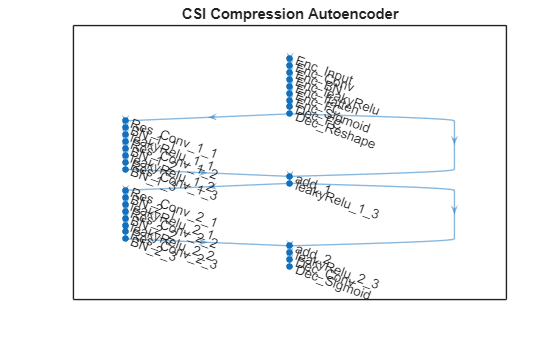#### Preprocess Channel Estimate

Preprocess the channel estimate to reduce the size and convert it to a real-valued array. This figure shows the channel estimate reduction preprocess.Assume that the channel coherence time is much larger than the slot time. Average the channel estimate over a slot and obtain a $\left[{\mathit{N}}_{\mathrm{subcarriers}}\text{\hspace{0.17em}}\text{\hspace{0.17em}}1\text{\hspace{0.17em}}{\mathit{N}}_{\mathrm{rx}}\text{\hspace{0.17em}}{\mathit{N}}_{\mathrm{tx}}\right]$array.

Hmean = mean(Hest,2);

To enable operation on subcarriers and Tx antennas, move the Tx and Rx antenna dimensions to the second and third dimensions, respectively.

Hmean = permute(Hmean,[1 4 3 2]);

To obtain the delay-angle representation of the channel, apply a 2-D discrete Fourier transform (DFT) over subcarriers and Tx antennas for each Rx antenna and slot. To demonstrate the workflow and reduce runtime, this subsection processes Rx channel 1 only.

Hdft2 = fft2(Hmean(:,:,1));

Since the multipath delay in the channel is limited, truncate the delay dimension to remove values that do not carry information. The sampling period on the delay dimension is ${T}_{delay}=1/\left({N}_{subcarriers}*{F}_{ss}\right)$, where ${F}_{ss}$ is subcarrier spacing. The expected RMS delay spread in delay samples is ${\tau }_{RMS}/{T}_{delay}$, where ${\tau }_{RMS}$ is the RMS delay spread of the channel in seconds.

Tdelay = 1/(autoEncOpt.NumSubcarriers*carrier.SubcarrierSpacing*1e3); rmsTauSamples = channel.DelaySpread / Tdelay; maxTruncationFactor = floor(autoEncOpt.NumSubcarriers / rmsTauSamples);

Truncate the channel estimate to an even number of samples that is 10 times the expected RMS delay spread. Increasing the truncationFactor value can decrease the performance loss due to preprocessing. But, doing so increases the neural network complexity, number of required training data points, and training time. A neural network with more learnable parameters might not converge to a better solution.

truncationFactor =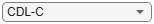10; maxDelay = round((channel.DelaySpread/Tdelay)*truncationFactor/2)*2
maxDelay = 28 
autoEncOpt.MaxDelay = maxDelay;

Calculate the truncation indices and truncate the channel estimate.

midPoint = floor(nSub/2); lowerEdge = midPoint - (nSub-maxDelay)/2 + 1; upperEdge = midPoint + (nSub-maxDelay)/2; Htemp = Hdft2([1:lowerEdge-1 upperEdge+1:end],:);

To get back to the subcarriers-Tx antennas domain, apply a 2-D inverse discrete Fourier transform (IDFT) to the truncated array . This process effectively decimates the channel estimate in the subcarrier axis.

Htrunc = ifft2(Htemp);

Separate the real and imaginary parts of the channel estimate to obtain a $\left[{\mathit{N}}_{\mathrm{delay}}\text{\hspace{0.17em}}\text{\hspace{0.17em}}{\mathit{N}}_{\mathrm{tx}}\text{\hspace{0.17em}}2\right]$ array.

HtruncReal = zeros(maxDelay,nTx,2); HtruncReal(:,:,1) = real(Htrunc); HtruncReal(:,:,2) = imag(Htrunc); %#ok<NASGU> 

Plot the channel estimate signal through the preprocessing steps. Images are scaled to help visualization.

plotPreprocessingSteps(Hmean(:,:,1),Hdft2,Htemp,Htrunc,nSub,nTx, ... maxDelay)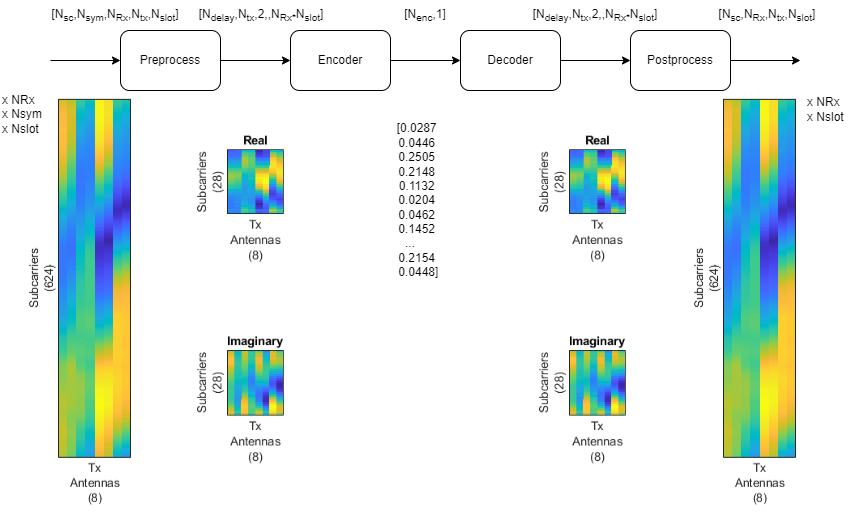#### Prepare Data in Bulk

The helperCSINetTrainingData helper function generates numTrainingChEst of preprocessed $\left[{\mathit{N}}_{\mathrm{delay}}\text{\hspace{0.17em}}\text{\hspace{0.17em}}{\mathit{N}}_{\mathrm{tx}}\text{\hspace{0.17em}}2\right]$ channel estimates by using the process described in this section. The function saves each $\left[{\mathit{N}}_{\mathrm{delay}}\text{\hspace{0.17em}}\text{\hspace{0.17em}}{\mathit{N}}_{\mathrm{tx}}\text{\hspace{0.17em}}2\right]$ channel estimate as an individual file in the dataDir with the prefix of trainingDataFilePrefix. If Parallel Computing Toolbox™ is available, helperCSINetTrainingData function uses parfor to parallelize data generation. Data generation takes less than three minutes on a PC with Intel® Xeon® W-2133 CPU @ 3.60GHz and running in parallel on six workers.

dataDir = fullfile(exRoot(),"Data"); trainingDataFilePrefix = "nr_channel_est"; if validateTrainingFiles(dataDir,trainingDataFilePrefix, ... numTrainingChEst,autoEncOpt,channel,carrier) == false disp("Starting training data generation") tic autoEncOpt.Normalization = false; % Do not normalize data yet helperCSINetTrainingData(dataDir,trainingDataFilePrefix, ... numTrainingChEst,carrier,channel,autoEncOpt); t = seconds(toc); t.Format = "hh:mm:ss"; disp(string(t) + " - Finished training data generation") end
Starting training data generation 
6 workers running 00:00:14 - 8% Completed 00:00:26 - 16% Completed 00:00:38 - 24% Completed 00:00:50 - 32% Completed 00:01:03 - 40% Completed 00:01:15 - 48% Completed 00:01:29 - 56% Completed 00:01:41 - 64% Completed 00:01:54 - 72% Completed 00:02:06 - 80% Completed 00:02:18 - 88% Completed 00:02:30 - 96% Completed 
00:02:37 - Finished training data generation 

Create a signalDatastore object to access the data. The signal datastore uses individual files for each data point.

sds = signalDatastore( ... fullfile(dataDir,"processed",trainingDataFilePrefix+"_*"));

Load data into memory, calculate the mean value and standard deviation, and then use the mean and standard deviation values to normalize the data.

HtruncRealCell = readall(sds); HtruncReal = cat(4,HtruncRealCell{:}); meanVal = mean(HtruncReal,'all')
meanVal = single -0.0236 
stdVal = std(HtruncReal,[],'all')
stdVal = single 16.0657 

Separate the data into training, validation, and test sets. Also, normalize the data to achieve zero mean and a target standard deviation of 0.0212, which restricts most of the data to the range of [-0.5 0.5].

N = size(HtruncReal, 4); numTrain = floor(N*10/15)
numTrain = 10000 
numVal = floor(N*3/15)
numVal = 3000 
numTest = floor(N*2/15)
numTest = 2000 
targetStd = 0.0212; HTReal = (HtruncReal(:,:,:,1:numTrain)-meanVal) ... /stdVal*targetStd+0.5; HVReal = (HtruncReal(:,:,:,numTrain+(1:numVal))-meanVal) ... /stdVal*targetStd+0.5; HTestReal = (HtruncReal(:,:,:,numTrain+numVal+(1:numTest))-meanVal) ... /stdVal*targetStd+0.5; autoEncOpt.MeanVal = meanVal; autoEncOpt.StdValue = stdVal; autoEncOpt.TargetSTDValue = targetStd; %#ok<STRNU> 

### Define and Train Neural Network Model

The second step of designing an AI-based system is to define and train the neural network model.

#### Define Neural Network

This example uses a modified version of the autoencoder neural network proposed in .

inputSize = [maxDelay nTx 2]; % Third dimension is real and imaginary parts nLinear = prod(inputSize); nEncoded = 64; autoencoderLGraph = layerGraph([ ... % Encoder imageInputLayer(inputSize,"Name","Htrunc", ... "Normalization","none","Name","Enc_Input") convolution2dLayer([3 3],2,"Padding","same","Name","Enc_Conv") batchNormalizationLayer("Epsilon",0.001,"MeanDecay",0.99, ... "VarianceDecay",0.99,"Name","Enc_BN") leakyReluLayer(0.3,"Name","Enc_leakyRelu") flattenLayer("Name","Enc_flatten") fullyConnectedLayer(nEncoded,"Name","Enc_FC") sigmoidLayer("Name","Enc_Sigmoid") % Decoder fullyConnectedLayer(nLinear,"Name","Dec_FC") functionLayer(@(x)dlarray(reshape(x,maxDelay,nTx,2,[]),'SSCB'), ... "Formattable",true,"Acceleratable",true,"Name","Dec_Reshape") ]); autoencoderLGraph = ... helperCSINetAddResidualLayers(autoencoderLGraph, "Dec_Reshape"); autoencoderLGraph = addLayers(autoencoderLGraph, ... [convolution2dLayer([3 3],2,"Padding","same","Name","Dec_Conv") ... sigmoidLayer("Name","Dec_Sigmoid") ... regressionLayer("Name","Dec_Output")]); autoencoderLGraph = ... connectLayers(autoencoderLGraph,"leakyRelu_2_3","Dec_Conv"); figure plot(autoencoderLGraph) title('CSI Compression Autoencoder')#### Train Neural Network

Set the training options for the autoencoder neural network and train the network using the trainNetwork (Deep Learning Toolbox) function. Training takes less than 15 minutes on an AMD EPYC 7262 3.2 GHz 8C/16T with 8 NVIDIA RTX A5000 GPUs with ExecutionEnvironment set to 'multi-gpu'. Set trainNow to false to load the pretrained network.

trainNow =false; miniBatchSize = 1000; options = trainingOptions("adam", ... InitialLearnRate=0.0074, ... LearnRateSchedule="piecewise", ... LearnRateDropPeriod=112, ... LearnRateDropFactor=0.6085, ... Epsilon=1e-7, ... MaxEpochs=1000, ... MiniBatchSize=miniBatchSize, ... Shuffle="every-epoch", ... ValidationData={HVReal,HVReal}, ... ValidationFrequency=20, ... Verbose=false, ... ValidationPatience=20, ... OutputNetwork="best-validation-loss", ... ExecutionEnvironment="auto", ... Plots='training-progress') %#ok<NASGU> 
options = TrainingOptionsADAM with properties: GradientDecayFactor: 0.9000 SquaredGradientDecayFactor: 0.9990 Epsilon: 1.0000e-07 InitialLearnRate: 0.0074 LearnRateSchedule: 'piecewise' LearnRateDropFactor: 0.6085 LearnRateDropPeriod: 112 L2Regularization: 1.0000e-04 GradientThresholdMethod: 'l2norm' GradientThreshold: Inf MaxEpochs: 1000 MiniBatchSize: 1000 Verbose: 0 VerboseFrequency: 50 ValidationData: {[28×8×2×3000 single] [28×8×2×3000 single]} ValidationFrequency: 20 ValidationPatience: 20 Shuffle: 'every-epoch' CheckpointPath: '' CheckpointFrequency: 1 CheckpointFrequencyUnit: 'epoch' ExecutionEnvironment: 'auto' WorkerLoad: [] OutputFcn: [] Plots: 'training-progress' SequenceLength: 'longest' SequencePaddingValue: 0 SequencePaddingDirection: 'right' DispatchInBackground: 0 ResetInputNormalization: 1 BatchNormalizationStatistics: 'population' OutputNetwork: 'best-validation-loss' 
if trainNow [net,trainInfo] = ... trainNetwork(HTReal,HTReal,autoencoderLGraph,options); %#ok<UNRCH> save("csiTrainedNetwork_" ... + string(datetime("now","Format","dd_MM_HH_mm")), ... 'net','trainInfo','options','autoEncOpt') else helperCSINetDownloadData() load("csiTrainedNetwork",'net','trainInfo','options','autoEncOpt') end
Starting download of data files from: https://www.mathworks.com/supportfiles/spc/CSI/TrainedCSIFeedbackAutoencoder_v22b1.tar Download complete. Extracting files. Extract complete. 

### Test Trained Network

Use the predict (Deep Learning Toolbox) function to process the test data.

HTestRealHat = predict(net,HTestReal);

Calculate the correlation and normalized mean squared error (NMSE) between the input and output of the autoencoder network. The correlation is defined as

$\rho =\mathbb{E}\left\{\frac{1}{N}\sum _{n=1}^{N}\frac{|{\underset{}{\overset{ˆ}{h}}}_{n}^{H}{h}_{n}|}{‖{\underset{}{\overset{ˆ}{h}}}_{n}{‖}_{2}‖{h}_{n}{‖}_{2}}\right\}$

where ${h}_{n}$ is the channel estimate at the input of the autoencoder and ${\underset{}{\overset{ˆ}{h}}}_{n}$ is the channel estimate at the output of the autoencoder. NMSE is defined as

$NMSE=\mathbb{E}\left\{\frac{{‖H-\underset{}{\overset{ˆ}{H}}‖}_{2}^{2}}{{‖H‖}_{2}^{2}}\right\}$

where $H$ is the channel estimate at the input of the autoencoder and $\underset{}{\overset{ˆ}{H}}$ is the channel estimate at the output of the autoencoder.

rho = zeros(numTest,1); nmse = zeros(numTest,1); for n=1:numTest in = HTestReal(:,:,1,n) + 1i*(HTestReal(:,:,2,n)); out = HTestRealHat(:,:,1,n) + 1i*(HTestRealHat(:,:,2,n)); % Calculate correlation n1 = sqrt(sum(conj(in).*in,'all')); n2 = sqrt(sum(conj(out).*out,'all')); aa = abs(sum(conj(in).*out,'all')); rho(n) = aa / (n1*n2); % Calculate NMSE mse = mean(abs(in-out).^2,'all'); nmse(n) = 10*log10(mse / mean(abs(in).^2,'all')); end figure tiledlayout(2,1) nexttile histogram(rho,"Normalization","probability") grid on title(sprintf("Autoencoder Correlation (Mean \\rho = %1.5f)", ... mean(rho))) xlabel("\rho"); ylabel("PDF") nexttile histogram(nmse,"Normalization","probability") grid on title(sprintf("Autoencoder NMSE (Mean NMSE = %1.2f dB)",mean(nmse))) xlabel("NMSE (dB)"); ylabel("PDF")### End-to-End CSI Feedback System

This figure shows the end-to-end processing of channel estimates for CSI feedback. The UE uses the CSI-RS signal to estimate the channel response for one slot, ${H}_{est}$. The preprocessed channel estimate, ${H}_{tr}$, is encoded by using the encoder portion of the autoencoder to produce a 1-by-${N}_{enc}$ compressed array. The compressed array is decompressed by the decoder portion of the autoencoder to obtain $\underset{}{\overset{ˆ}{{H}_{tr}}}$. Postprocessing $\underset{}{\overset{ˆ}{{H}_{tr}}}$ produces $\underset{}{\overset{ˆ}{{H}_{est}}}$.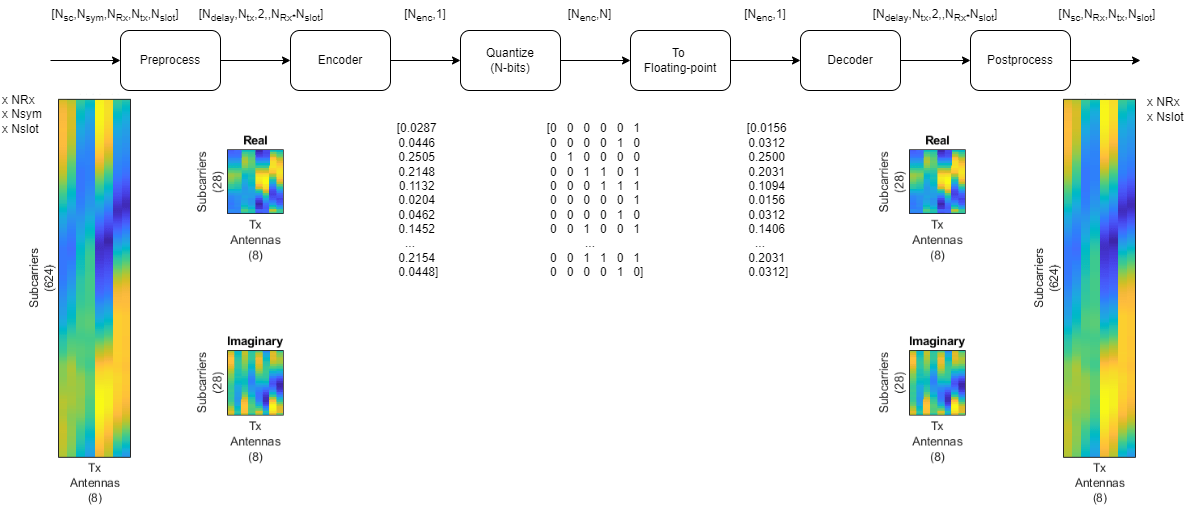To obtain the encoded array, split the autoencoder into two parts: the encoder network and the decoder network.

[encNet,decNet] = helperCSINetSplitEncoderDecoder(net,"Enc_Sigmoid"); plotNetwork(net,encNet,decNet)Generate channel estimates.

nSlots = 100; Hest = helperCSINetChannelEstimate(nSlots,carrier,channel);

Encode and decode the channel estimates with Normalization set to true.

autoEncOpt.Normalization = true; codeword = helperCSINetEncode(encNet, Hest, autoEncOpt); Hhat = helperCSINetDecode(decNet, codeword, autoEncOpt);

Calculate the correlation and NMSE for the end-to-end CSI feedback system.

H = squeeze(mean(Hest,2)); rhoE2E = zeros(nRx,nSlots); nmseE2E = zeros(nRx,nSlots); for rx=1:nRx for n=1:nSlots out = Hhat(:,rx,:,n); in = H(:,rx,:,n); rhoE2E(rx,n) = helperCSINetCorrelation(in,out); nmseE2E(rx,n) = helperNMSE(in,out); end end figure tiledlayout(2,1) nexttile histogram(rhoE2E,"Normalization","probability") grid on title(sprintf("End-to-End Correlation (Mean \\rho = %1.5f)", ... mean(rhoE2E,'all'))) xlabel("\rho"); ylabel("PDF") nexttile histogram(nmseE2E,"Normalization","probability") grid on title(sprintf("End-to-End NMSE (Mean NMSE = %1.2f dB)", ... mean(nmseE2E,'all'))) xlabel("NMSE (dB)"); ylabel("PDF")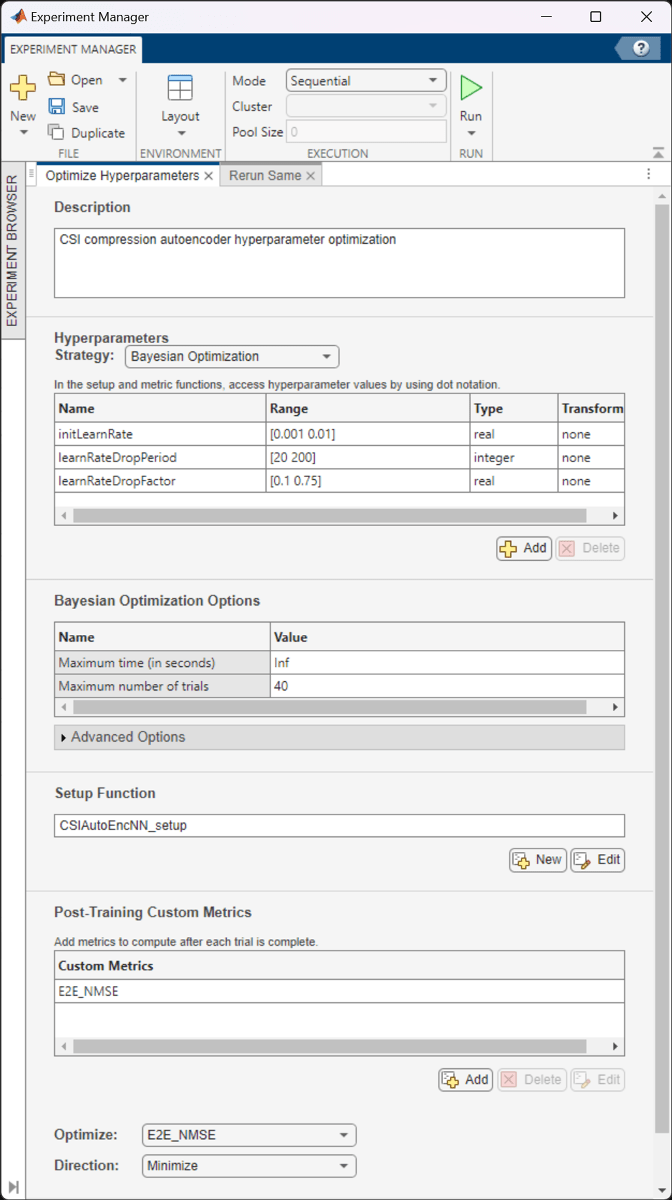### Effect of Quantized Codewords

Practical systems require quantizing the encoded codeword by using a small number of bits. Simulate the effect of quantization across the range of [2, 10] bits. The results show that 6-bits is enough to closely approximate the single-precision performance.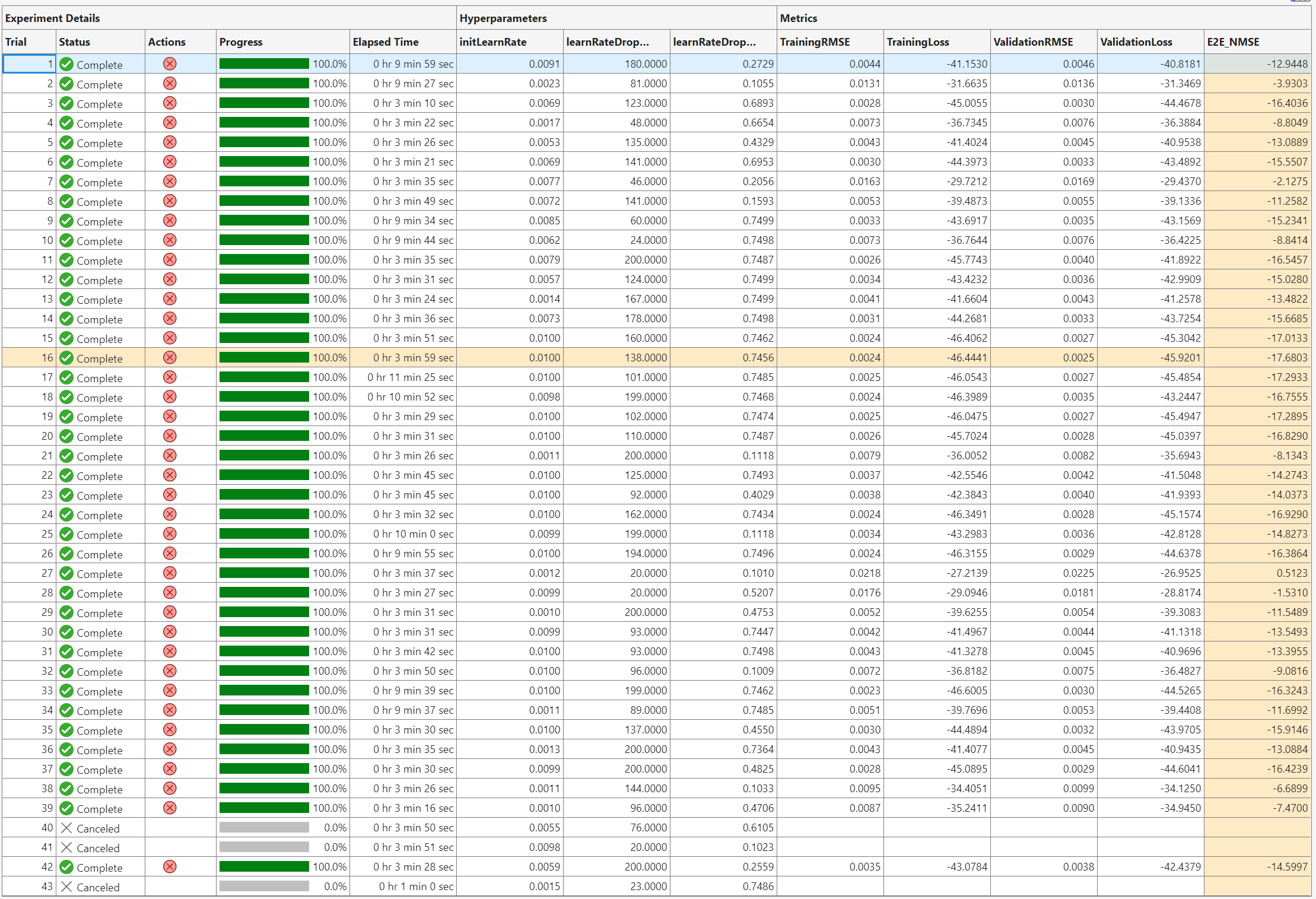maxVal = 1; minVal = -1; idxBits = 1; nBitsVec = 2:10; rhoQ = zeros(nRx,nSlots,length(nBitsVec)); nmseQ = zeros(nRx,nSlots,length(nBitsVec)); for numBits = nBitsVec disp("Running for " + numBits + " bit quantization") % Quantize between 0:2^n-1 to get bits qCodeword = uencode(double(codeword*2-1), numBits); % Get back the floating point, quantized numbers codewordRx = (single(udecode(qCodeword,numBits))+1)/2; Hhat = helperCSINetDecode(decNet, codewordRx, autoEncOpt); H = squeeze(mean(Hest,2)); for rx=1:nRx for n=1:nSlots out = Hhat(:,rx,:,n); in = H(:,rx,:,n); rhoQ(rx,n,idxBits) = helperCSINetCorrelation(in,out); nmseQ(rx,n,idxBits) = helperNMSE(in,out); end end idxBits = idxBits + 1; end
Running for 2 bit quantization Running for 3 bit quantization Running for 4 bit quantization Running for 5 bit quantization Running for 6 bit quantization Running for 7 bit quantization Running for 8 bit quantization Running for 9 bit quantization Running for 10 bit quantization 
figure tiledlayout(2,1) nexttile plot(nBitsVec,squeeze(mean(rhoQ,[1 2])),'*-') title("Correlation (Codeword-" + size(codeword,3) + ")") xlabel("Number of Quantization Bits"); ylabel("\rho") grid on nexttile plot(nBitsVec,squeeze(mean(nmseQ,[1 2])),'*-') title("NMSE (Codeword-" + size(codeword,3) + ")") xlabel("Number of Quantization Bits"); ylabel("NMSE (dB)") grid on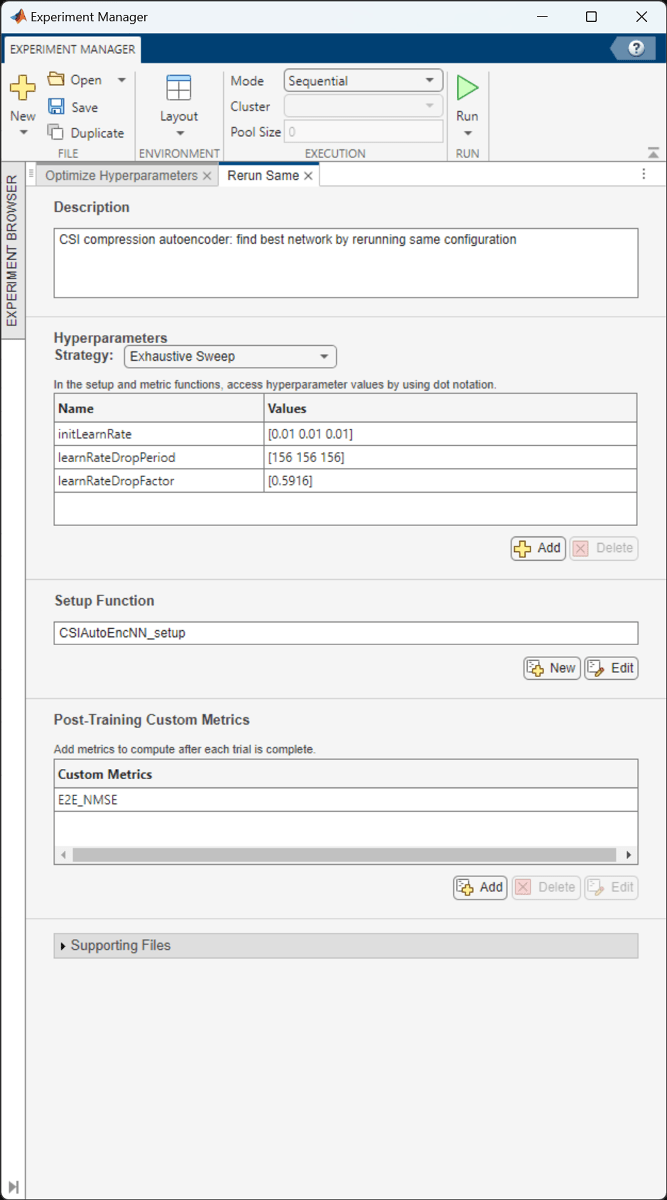### Further Exploration

The autoencoder is able to compress a [624 8] single-precision complex channel estimate array into a [64 1] single-precision array with a mean correlation factor of 0.99 and an NMSE of –16 dB. Using 6-bit quantization requires only 384 bits of CSI feedback data, which equates to a compression ratio of approximately 800:1.

display("Compression ratio is " + (624*8*32*2)/(64*6) + ":" + 1)
 "Compression ratio is 832:1" 

Investigate the effect of truncationFactor on the system performance. Vary the 5G system parameters, channel parameters, and number of encoded symbols and then find the optimum values for the defined channel.

The NR PDSCH Throughput Using Channel State Information Feedback (5G Toolbox) example shows how to use channel state information (CSI) feedback to adjust the physical downlink shared channel (PDSCH) parameters and measure throughput. Replace the CSI feedback algorithm with the CSI compression autoencoder and compare performance.

#### Helper Functions

Explore the helper functions to see the detailed implementation of the system.

#### Training Data Generation

helperCSINetChannelEstimate

helperCSINetTrainingData

#### Network Definition and Manipulation

helperCSINetLayerGraph

helperCSINetSplitEncoderDecoder

#### CSI Processing

helperCSINetPreprocessChannelEstimate

helperCSINetPostprocessChannelEstimate

helperCSINetEncode

helperCSINetDecode

#### Performance Measurement

helperCSINetCorrelation

helperNMSE

### Appendix: Optimize Hyperparameters with Experiment Manager

Use the Experiment Manager app to find the optimal parameters. CSITrainingProject.mlproj is a preconfigured project. Extract the project.

if ~exist("CSITrainingProject","dir") projRoot = helperCSINetExtractProject(); else projRoot = fullfile(exRoot(),"CSITrainingProject"); end

To open the project, start the Experiment Manager app and open the following file.

disp(fullfile(".","CSITrainingProject","CSITrainingProject.prj"))
.\CSITrainingProject\CSITrainingProject.prj 

The Optimize Hyperparameters experiment uses Bayesian optimization with hyperparameter search ranges specified as in the following figure. The experiment setup function is CSIAutoEncNN_setup. The custom metric function is NMSE.The optimal parameters are 0.0074 for initial learning rate, 112 iterations for the learning rate drop period, and 0.6085 for learning rate drop factor. After finding the optimal hyperparameters, train the network with same parameters multiple times to find the best trained network. Increase the maximum iterations by a factor of two.The sixth trial produced the best NMSE. This example uses this trained network as the saved network.#### Configuring Batch Mode

When execution Mode is set to Batch Sequential or Batch Simultaneous, training data must be accessible to the workers in a location defined by the dataDir variable in the Prepare Data in Bulk section. Set dataDir to a network location that is accessible by the workers. For more information, see Offload Experiments as Batch Jobs to Cluster (Deep Learning Toolbox).

### Local Functions

function plotChannelResponse(Hest) %plotChannelResponse Plot channel response figure tiledlayout(2,2) nexttile waterfall(abs(Hest(:,:,1,1))') xlabel("Subcarriers"); ylabel("Symbols"); zlabel("Channel Magnitude") view(15,30) colormap("cool") title("Rx=1, Tx=1") nexttile plot(squeeze(abs(Hest(:,1,:,1)))) grid on xlabel("Subcarriers"); ylabel("Channel Magnitude") legend("Rx 1", "Rx 2") title("Symbol=1, Tx=1") nexttile waterfall(squeeze(abs(Hest(:,1,1,:)))') view(-45,75) grid on xlabel("Subcarriers"); ylabel("Tx"); zlabel("Channel Magnitude") title("Symbol=1, Rx=1") nexttile plot(squeeze(abs(Hest(400,1,:,:)))') grid on xlabel("Tx"); ylabel("Channel Magnitude") legend("Rx 1", "Rx 2") title("Subcarrier=400, Symbol=1") end function valid = validateTrainingFiles(dataDir,filePrefix,expN, ... opt,channel,carrier) %validateTrainingFiles Validate training data files % V = validateTrainingFiles(DIR,PRE,N,OPT,CH,CR) checks the DIR directory % for training data files with a prefix of PRE. It checks if there are % N*OPT.NumRxAntennas files, channel configuration is same as CH, and % carrier configuration is same as CR. valid = true; files = dir(fullfile(dataDir,filePrefix+"*")); if isempty(files) valid = false; return end if exist(fullfile(dataDir,"info.mat"),"file") infoStr = load(fullfile(dataDir,"info.mat")); if ~isequal(get(infoStr.channel),get(channel)) ... || ~isequal(infoStr.carrier,carrier) valid = false; end else valid = false; end if valid valid = (expN <= (length(files)*opt.NumRxAntennas)); % Check size of Hest in the files load(fullfile(files(1).folder,files(1).name),'H') if ~isequal(size(H),[opt.NumSubcarriers opt.NumSymbols ... opt.NumRxAntennas opt.NumTxAntennas]) valid = false; end end if ~valid || expN < (length(files)*opt.NumRxAntennas) disp("Removing invalid data directory: " + files(1).folder) rmdir(files(1).folder,'s') end end function plotNetwork(net,encNet,decNet) %plotNetwork Plot autoencoder network % plotNetwork(NET,ENC,DEC) plots the full autoencoder network together % with encoder and decoder networks. fig = figure; t1 = tiledlayout(1,2,'TileSpacing','Compact'); t2 = tiledlayout(t1,1,1,'TileSpacing','Tight'); t3 = tiledlayout(t1,2,1,'TileSpacing','Tight'); t3.Layout.Tile = 2; nexttile(t2) plot(net) title("Autoencoder") nexttile(t3) plot(encNet) title("Encoder") nexttile(t3) plot(decNet) title("Decoder") pos = fig.Position; pos(3) = pos(3) + 200; pos(4) = pos(4) + 300; pos(2) = pos(2) - 300; fig.Position = pos; end function plotPreprocessingSteps(Hmean,Hdft2,Htemp,Htrunc, ... nSub,nTx,maxDelay) %plotPreprocessingSteps Plot preprocessing workflow hfig = figure; hfig.Position(3) = hfig.Position(3)*2; subplot(2,5,[1 6]) himg = imagesc(abs(Hmean)); himg.Parent.YDir = "normal"; himg.Parent.Position(3) = 0.05; himg.Parent.XTick=''; himg.Parent.YTick=''; xlabel(sprintf('Tx\nAntennas\n(%d)',nTx)); ylabel(sprintf('Subcarriers\n(%d)',nSub')); title("Measured") subplot(2,5,[2 7]) himg = image(abs(Hdft2)); himg.Parent.YDir = "normal"; himg.Parent.Position(3) = 0.05; himg.Parent.XTick=''; himg.Parent.YTick=''; title("2-D DFT") xlabel(sprintf('Tx\nAngle\n(%d)',nTx)); ylabel(sprintf('Delay Samples\n(%d)',nSub')); subplot(2,5,[3 8]) himg = image(abs(Htemp)); himg.Parent.YDir = "normal"; himg.Parent.Position(3) = 0.05; himg.Parent.Position(4) = himg.Parent.Position(4)*10*maxDelay/nSub; himg.Parent.Position(2) = (1 - himg.Parent.Position(4)) / 2; himg.Parent.XTick=''; himg.Parent.YTick=''; xlabel(sprintf('Tx\nAngle\n(%d)',nTx)); ylabel(sprintf('Delay Samples\n(%d)',maxDelay')); title("Truncated") subplot(2,5,[4 9]) himg = imagesc(abs(Htrunc)); himg.Parent.YDir = "normal"; himg.Parent.Position(3) = 0.05; himg.Parent.Position(4) = himg.Parent.Position(4)*10*maxDelay/nSub; himg.Parent.Position(2) = (1 - himg.Parent.Position(4)) / 2; himg.Parent.XTick=''; himg.Parent.YTick=''; xlabel(sprintf('Tx\nAntennas\n(%d)',nTx)); ylabel(sprintf('Subcarriers\n(%d)',maxDelay')); title("2-D IDFT") subplot(2,5,5) himg = imagesc(real(Htrunc)); himg.Parent.YDir = "normal"; himg.Parent.Position(3) = 0.05; himg.Parent.Position(4) = himg.Parent.Position(4)*10*maxDelay/nSub; himg.Parent.Position(2) = himg.Parent.Position(2) + 0.18; himg.Parent.XTick=''; himg.Parent.YTick=''; xlabel(sprintf('Tx\nAntennas\n(%d)',nTx)); ylabel(sprintf('Subcarriers\n(%d)',maxDelay')); title("Real") subplot(2,5,10) himg = imagesc(imag(Htrunc)); himg.Parent.YDir = "normal"; himg.Parent.Position(3) = 0.05; himg.Parent.Position(4) = himg.Parent.Position(4)*10*maxDelay/nSub; himg.Parent.Position(2) = himg.Parent.Position(2) + 0.18; himg.Parent.XTick=''; himg.Parent.YTick=''; xlabel(sprintf('Tx\nAntennas\n(%d)',nTx)); ylabel(sprintf('Subcarriers\n(%d)',maxDelay')); title("Imaginary") end function rootDir = exRoot() %exRoot Example root directory rootDir = fileparts(which("helperCSINetLayerGraph")); end

### References

 Wen, Chao-Kai, Wan-Ting Shih, and Shi Jin. “Deep Learning for Massive MIMO CSI Feedback.” IEEE Wireless Communications Letters 7, no. 5 (October 2018): 748–51. https://doi.org/10.1109/LWC.2018.2818160.

 Zimaglia, Elisa, Daniel G. Riviello, Roberto Garello, and Roberto Fantini. “A Novel Deep Learning Approach to CSI Feedback Reporting for NR 5G Cellular Systems.” In 2020 IEEE Microwave Theory and Techniques in Wireless Communications (MTTW), 47–52. Riga, Latvia: IEEE, 2020. https://doi.org/10.1109/MTTW51045.2020.9245055.